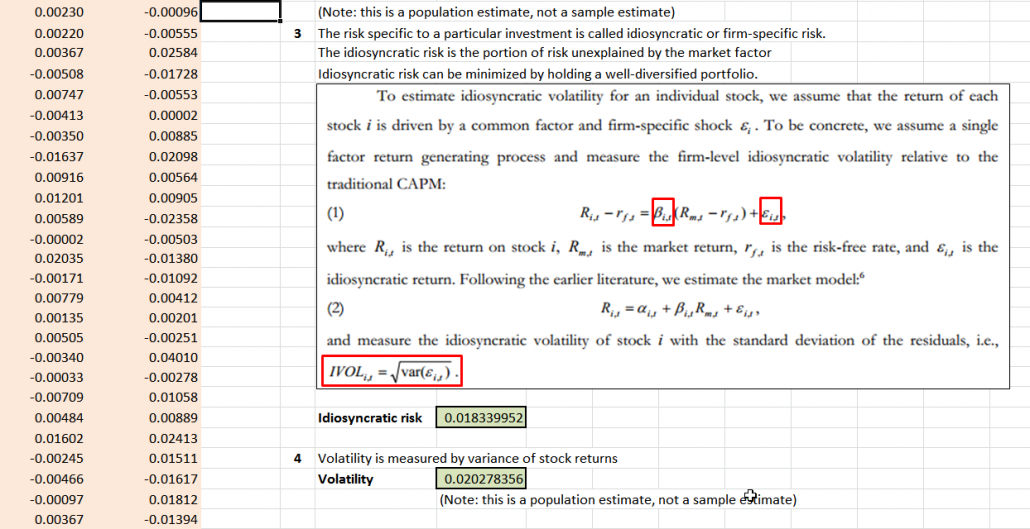Wild-swinging oil prices have caused some chaos, or “volatility,” in the financial markets recently. We’ve also heard a lot in the financial media regarding the strong performance of “low volatility” funds.
But what exactly is “volatility” and how do we measure it?
We’ve posted some thoughts on the low volatility anomaly (e.g. Avoid High Beta Stocks. Period.)
But the use of volatility is somewhat ambiguous.
In the studies we’ve conducted we’ve referenced  “beta” and Idiosyncratic volatility (“ivol”).
We thought it might make sense to take a quick break and describe how these concepts are calculated. We first describe 3 ways in which we can describe “volatility” and then we provide a spreadsheet so you can see these calculations in action.
Standard Deviation:
When we talk about a security’s volatility, we first think of the “standard deviation” of stock returns, which measures the degree of fluctuations in relation to its mean return over a period of time. This measure is calculated independently of the market and only requires data on the stock.
BETA:
While Standard Deviation measures the disparity of a security’s return over a period of time, “BETA”, another widely used metric, measures the co-movement of this security with the market. BETA can be calculated by regressing daily stock returns on a market benchmark (such as value weighted CRSP) over a period of time.
Idiosyncratic volatility (IVOL):
Business school professors tell us that there are 2 types of risk: systematic risk and unsystematic risk. Systematic risk can be estimated by Beta. The idiosyncratic risk is the portion of risk that unexplained by BETA. We calculate Idiosyncratic volatility (IVOL) as the standard deviation of the residuals from a regression that uses Beta to estimate the relationship between a given asset and the market.
Calculation Example:

1. We use Amazon (Ticker: AMZN) stock as a single stock example, and use the value weighted CRSP index as the market benchmark. If forming a portfolio on 1/31/13, we would use daily returns from 1/31/12-1/31/13 to calculate beta and IVOL. (We use daily data to improve frequency and accuracy.)
2. BETA calculation follows the basic methodology in Betting Against Beta.
3. IVOL calculation follows the basic methodology in IVOL and the Cross-Section of Expected Returns.